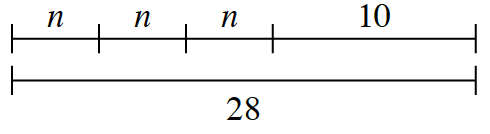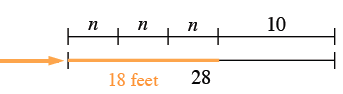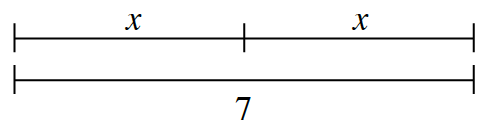### Home > CC2MN > Chapter 3 > Lesson 3.2.2 > Problem3-48

3-48.

Each of the diagrams below represents tightrope moves for an acrobat. For each diagram:

• Describe what the diagram shows about the routine and the length of the tightrope.

• Figure out the length of the acrobat’s trick.

1.This is similar to writing an expression for an acrobat's routine. Think of the tricks as being numerical lengths of the rope.

First, describe the routine based on the diagram.

The acrobat performs a trick three times and then walks $10$ feet to get to the end of the $28$ foot tight rope.

Next, determine how far each trick travels. Looking at the tightropes, the $3$ $n$'s match up with $18$ feet. Since $3$ unknown, but equal, lengths combine to make $18$ feet, you know that each length is $6$ feet.The trick travels $6$ feet.

2.Follow the same steps as in part (a).